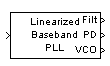Documentation

# Linearized Baseband PLL

Implement linearized version of baseband phase-locked loop

## Library

Components sublibrary of Synchronization## Description

The Linearized Baseband PLL block is a feedback control system that automatically adjusts the phase of a locally generated signal to match the phase of an input signal. Unlike the Phase-Locked Loop block, this block uses a baseband model method. Unlike the Baseband PLL block, which uses a nonlinear model, this block simplifies the computations by using x to approximate sin(x). The baseband PLL model depends on the amplitude of the incoming signal but does not depend on a carrier frequency.

This PLL has these three components:

• An integrator used as a phase detector.

• A filter. You specify the filter's transfer function using the Lowpass filter numerator and Lowpass filter denominator parameters. Each is a vector that gives the respective polynomial's coefficients in order of descending powers of s.

To design a filter, you can use functions such as `butter`, `cheby1`, and `cheby2` in Signal Processing Toolbox™ software. The default filter is a Chebyshev type II filter whose transfer function arises from the command below.

`[num, den] = cheby2(3,40,100,'s')`

• A voltage-controlled oscillator (VCO). You specify the sensitivity of the VCO signal to its input using the VCO input sensitivity parameter. This parameter, measured in Hertz per volt, is a scale factor that determines how much the VCO shifts from its quiescent frequency.

This block accepts a sample-based scalar input signal. The input signal represents the received signal. The three output ports produce:

• The output of the filter

• The output of the phase detector

• The output of the VCO

## Parameters

Lowpass filter numerator

The numerator of the lowpass filter transfer function, represented as a vector that lists the coefficients in order of descending powers of s.

Lowpass filter denominator

The denominator of the lowpass filter transfer function, represented as a vector that lists the coefficients in order of descending powers of s.

VCO input sensitivity (Hz/V)

This value scales the input to the VCO and, consequently, the shift from the VCO's quiescent frequency.

## References

For more information about phase-locked loops, see the works listed in Selected Bibliography for Synchronization.